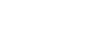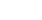# 美国留学网，留学美国，美国留学美国留学服务协会会员教育部资质认证：BJ2000016客服电话：400-609-1118

SSAT数学 Fractions练习题

1. There are 8 ounces in a 1/2 pound. How many ounces are in 7 3/4 lbs?
A. 12 ounces
B. 86 ounces
C. 119 ounces
D. 124 ounces
E. 138 ounces
2. If the value of x and y in the following fraction are both tripled, how does the value of the fraction change? XZ Y
A. increases by half
B. decreases by half
C. triples
D. doubles
E. remains the same
3. Which of the following fractions is the equivalent of 0.5%
A. 1/20
B. 1/200
C. 1/2000
D. 1/5
E. 1/500
4. Which of these numbers is a factor of 21
A. 2
B. 5
C. 7
D. 42
E. 44
5. If the average person drinks 8, (8oz) glasses of water per day, a person who drinks 12.8 oz of water after a morning exercise session has consumed what fraction of the daily average?
A. 1/3
B. 1/5
C. 1/7
D. 1/9
E. 1/10
6. You need 4/5 cups of water for a recipe. You accidentally put 1/3 cups into the mixing bowl with the dry ingredients. How much more water in cups do you need to add?
A. 1/3 cups
B. 2/3 cups
C. 1/15 cups
D. 7/15 cups
E.7/16 cups
7. ¾ - ½ =
A. ¼
B. 1/3
C. ½
D. 2/3
E. 2/5
8. 7 1/2 - 5 3/8 =
A. 1 1/2
B. 1 2/3
C. 2 1/8
D. 3 1/4
E. 3
1. (D) 2. (E) 3. (B) 4. (C) 5. (B) 6. (D) 7. (A) 8. (C)Offer案例D同学O同学D同学